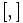# SmallGroup(128,1015)

View a complete list of particular groups (this is a very huge list!)[SHOW MORE]

## Definition

This is a group of order 128 given by the following presentation, where$e$ is used to denote the identity element:$G := \langle a_1, a_2, a_3, a_4 \mid a_1^2 = a_2^4 = a_3^4 = a_4^4 = e, [a_1,a_2] = a_3^2, [a_1,a_3] = e, [a_2,a_3] = a_4^2, [a_1,a_4] = [a_2,a_4] = [a_3,a_4] = e \rangle$

For the definition,$[ , ]$ stands for the commutator. It does not matter whether we choose the left or right action convention for the commutator -- the groups defined in both cases are isomorphic.

## Arithmetic functions

Want to compare and contrast arithmetic function values with other groups of the same order? Check out groups of order 128#Arithmetic functions

## GAP implementation

### Group ID

This finite group has order 128 and has ID 1015 among the groups of order 128 in GAP's SmallGroup library. For context, there are groups of order 128. It can thus be defined using GAP's SmallGroup function as:

SmallGroup(128,1015)

For instance, we can use the following assignment in GAP to create the group and name it$G$:

gap> G := SmallGroup(128,1015);

Conversely, to check whether a given group$G$ is in fact the group we want, we can use GAP's IdGroup function:

IdGroup(G) = [128,1015]

or just do:

IdGroup(G)

to have GAP output the group ID, that we can then compare to what we want.

### Description by presentation

gap> F := FreeGroup(4);
<free group on the generators [ f1, f2, f3, f4 ]>
gap> G := F/[F.1^2,F.2^4,F.3^4,F.4^4,Comm(F.1,F.2)*F.3^(-2),Comm(F.1,F.3),Comm(F.2,F.3)*F.4^(-2),Comm(F.1,F.4),Comm(F.2,F.4),Comm(F.3,F.4)];
<fp group on the generators [ f1, f2, f3, f4 ]>
gap> IdGroup(G);
[ 128, 1015 ]